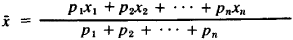# Weighted Results of Measurements

The following article is from The Great Soviet Encyclopedia (1979). It might be outdated or ideologically biased.

## Weighted Results of Measurements

numbers that express the relative accuracy of the results of measurements.

The weighted results of measurements are inversely proportional to the dispersions—that is, to the average values of the squares of the respective errors. The reliable establishment of weighted results of measurements in practice is especially important in those instances where measurements are made with varying degrees of precision. It is impossible to ignore these differences in using the results of similar measurements, since this would devalue the best measurements, placing them on the same level as those of little reliability. Therefore, greater weight is assigned to the more precise measurements. In the case of several independent measurements x1, x2, . . . , xn of the same quantity a, assuming that the measurements are free of systematic error and have the respective weights p1 p2, . . . , pn, the most reliable estimate of the quantity a is the following weighted mean:which has the weight p = p1 + p2+ . . . + pn Among all the linear estimates the weighted mean1 has the minimal dispersion.

### REFERENCE

Urbakh, V. Iu. Biometrieheskie metody [2nd ed.]. Moscow, 1964.
The Great Soviet Encyclopedia, 3rd Edition (1970-1979). © 2010 The Gale Group, Inc. All rights reserved.
Site: Follow: Share:
Open / Close